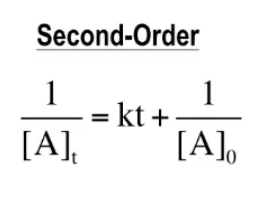# Problem: What is the half-life for the decomposition of O3 when the concentration of O3 is 2.35 × 10−6 M? The rate constant for this second-order reaction is 50.4 L/mol/h.

###### FREE Expert Solution

The integrated rate law for a second-order reaction is shown below:Where:

[A]t = amount at time t
[A]0 = initial amount
k = rate constant
t = time

85% (77 ratings)###### Problem Details

What is the half-life for the decomposition of O3 when the concentration of O3 is 2.35 × 10−6 M? The rate constant for this second-order reaction is 50.4 L/mol/h.